Publicité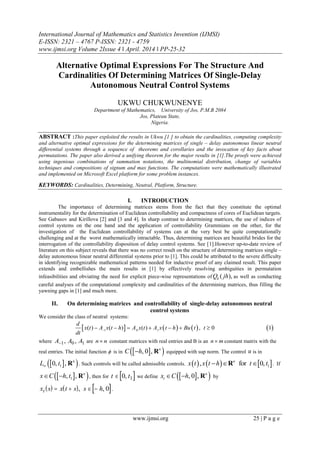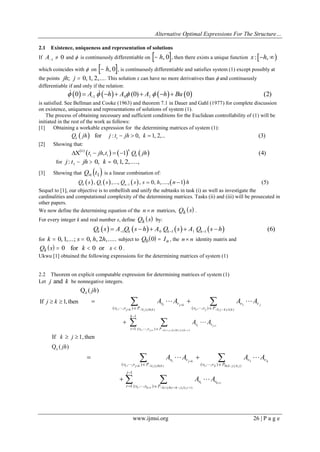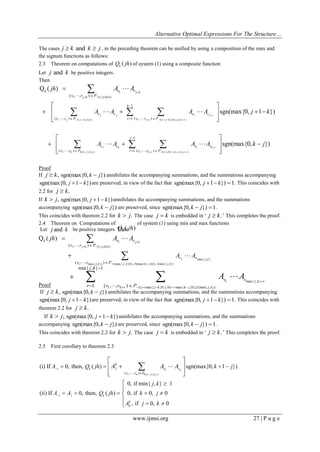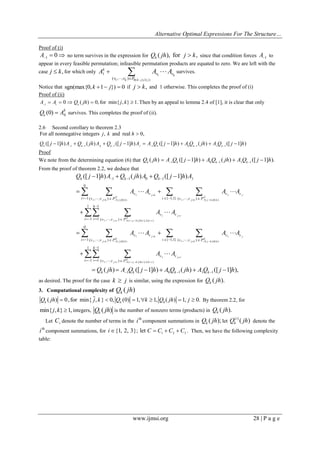Publicité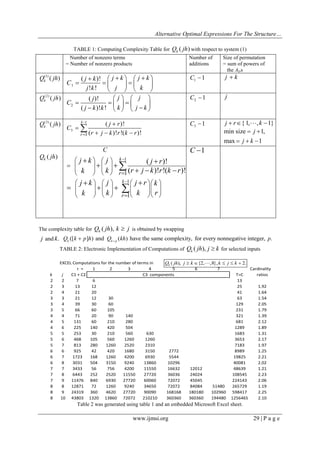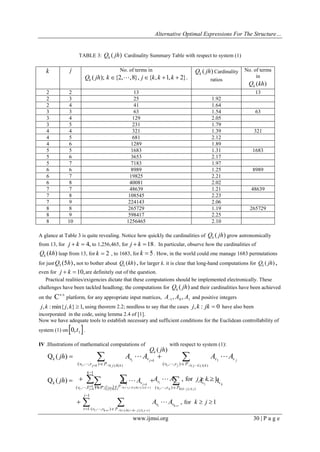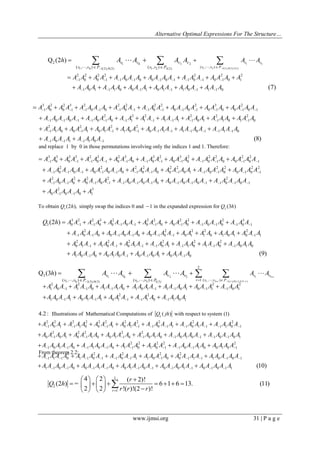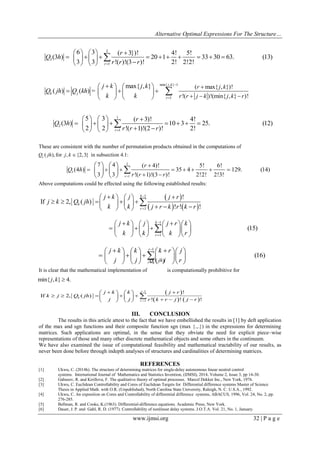Prochain SlideShare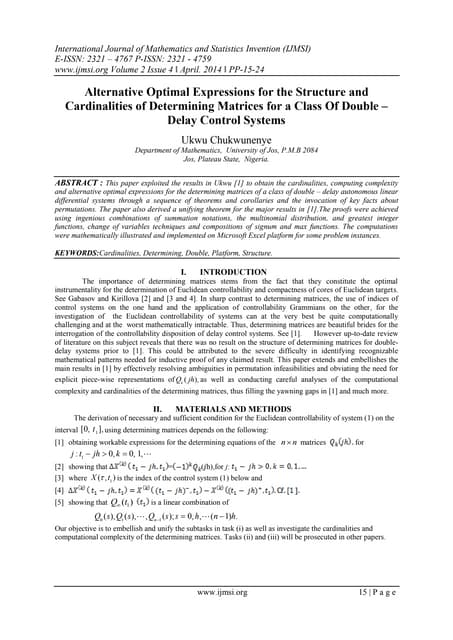C024015024
Chargement dans ... 3
1 sur 8
Publicité

### D024025032

1. International Journal of Mathematics and Statistics Invention (IJMSI) E-ISSN: 2321 – 4767 P-ISSN: 2321 - 4759 www.ijmsi.org Volume 2Issue 4 ǁ April. 2014 ǁ PP-25-32 www.ijmsi.org 25 | P a g e Alternative Optimal Expressions For The Structure And Cardinalities Of Determining Matrices Of Single-Delay Autonomous Neutral Control Systems UKWU CHUKWUNENYE Department of Mathematics, University of Jos, P.M.B 2084 Jos, Plateau State, Nigeria. ABSTRACT :This paper exploited the results in Ukwu [1 ] to obtain the cardinalities, computing complexity and alternative optimal expressions for the determining matrices of single – delay autonomous linear neutral differential systems through a sequence of theorems and corollaries and the invocation of key facts about permutations. The paper also derived a unifying theorem for the major results in .The proofs were achieved using ingenious combinations of summation notations, the multinomial distribution, change of variables techniques and compositions of signum and max functions. The computations were mathematically illustrated and implemented on Microsoft Excel platform for some problem instances. KEYWORDS: Cardinalities, Determining, Neutral, Platform, Structure. I. INTRODUCTION The importance of determining matrices stems from the fact that they constitute the optimal instrumentality for the determination of Euclidean controllability and compactness of cores of Euclidean targets. See Gabasov and Kirillova  and [3 and 4]. In sharp contrast to determining matrices, the use of indices of control systems on the one hand and the application of controllability Grammians on the other, for the investigation of the Euclidean controllability of systems can at the very best be quite computationally challenging and at the worst mathematically intractable. Thus, determining matrices are beautiful brides for the interrogation of the controllability disposition of delay control systems. See .However up-to-date review of literature on this subject reveals that there was no correct result on the structure of determining matrices single – delay autonomous linear neutral differential systems prior to . This could be attributed to the severe difficulty in identifying recognizable mathematical patterns needed for inductive proof of any claimed result. This paper extends and embellishes the main results in  by effectively resolving ambiguities in permutation infeasibilities and obviating the need for explicit piece-wise representations of ( ),kQ jh as well as conducting careful analyses of the computational complexity and cardinalities of the determining matrices, thus filling the yawning gaps in  and much more. II. On determining matrices and controllability of single-delay autonomous neutral control systems We consider the class of neutral systems:        1 0 1 ( ) ( ) ( ) , 0 1 d x t A x t h A x t A x t h Bu t t dt         where A A A1 0 1, , are n n constant matrices with real entries and B is an n m constant matrix with the real entries. The initial function  is in   , 0 , n C h R equipped with sup norm. The control u is in   10, , n L t R . Such controls will be called admissible controls.      1, for 0,n x t x t h t t  R . If   1, , n x C h t  R , then for  t t 0 1, we define   , 0 , n tx C h  R by      x s x t s s ht    , , 0 .
2. Alternative Optimal Expressions For The Structure… www.ijmsi.org 26 | P a g e 2.1 Existence, uniqueness and representation of solutions If A 1 0 and  is continuously differentiable on   h, 0 , then there exists a unique function  : ,x h  which coincides with  on   h, 0 , is continuously differentiable and satisfies system (1) except possibly at the points jh j; , , ,... 0 1 2 . This solution x can have no more derivatives than  and continuously differentiable if and only if the relation:        1 0 10 (0) 0 (2)A h A A h Bu          is satisfied. See Bellman and Cooke (1963) and theorem 7.1 in Dauer and Gahl (1977) for complete discussion on existence, uniqueness and representations of solutions of system (1). The process of obtaining necessary and sufficient conditions for the Euclidean controllability of (1) will be initiated in the rest of the work as follows:  Obtaining a workable expression for the determining matrices of system (1):   1 for : 0, 1, 2,.. (3)k Q jh j t jh k    Showing that:        1 1 , 1 (4) kk k t jh t Q jh    for j t jh k: , , , ,....,1 0 0 1 2    Showing that  Q t 1 is a linear combination of:        0 1 1 , ,..., , 0, ,...., 1 (5)n Q s Q s Q s s h n h   Sequel to , our objective is to embellish and unify the subtasks in task (i) as well as investigate the cardinalities and computational complexity of the determining matrices. Tasks (ii) and (iii) will be prosecuted in other papers. We now define the determining equation of the n n matrices,  Q sk . For every integer k and real number s, define  Q sk by:        1 0 1 1 1 (6)k k k kQ s A Q s h A Q s A Q s h       for k s h h 0 1 0 2, ,...; , , ,..... subject to  Q In0 0  , the n n identity matrix and  Q s k sk   0 0 0for or . Ukwu  obtained the following expressions for the determining matrices of system (1) 2.2 Theorem on explicit computable expression for determining matrices of system (1) Let andj k be nonnegative integers. If 1,thenj k  1 1 1( ),0( ),1( ) 1 1 1 11( ),0( ) 1( ),1( ) 1 1 ( , , ) ( , , ) ( , , ) Q ( ) j r j r r j k r k r jj k jj k j k j k k k k v v r v v P v v v v v v P v v P jh A A A A A A                              1 1 1 11( ),0( ) 0( ),1( ) 1 1 1( ),0( ),1( ) ( , , ) ( , , ) 1 1 ( , , ) If 1, then Q ( )k j k k j k j k k k j j k r k r r r k j j r v v v v v v P v v P j v v r v v P k j jh A A A A A A                              
3. Alternative Optimal Expressions For The Structure… www.ijmsi.org 27 | P a g e The cases andj k k j  , in the preceding theorem can be unified by using a composition of the max and the signum functions as follows: 2.3 Theorem on computations of ( )k Q jh of system (1) using a composite function Let andj k be positive integers. Then 1 1 1 1( ),1( ) 1 1( ),0( ),1( ) 1 1 1( ),0( ) 1 ( , , ) 1 ( , , ) ( , , ) Q ( ) sgn(max{0, 1 })j j r j j k k j r r j k r k r j k j k j k k k v v v v v v P r v v P v v v v P jh A A A A j k A A                                      1 1 1 0( ),1( ) 1 1( ),0( ),1( ) 1 ( , , ) 1 ( , , ) sgn(max{0, })k k r k k j j k r r r k j j r j v v v v v v P r v v P A A A A k j                          Proof If ,j k sgn(max{0, })k j annihilates the accompanying summations, and the summations accompanying sgn(max{0, 1 })j k  are preserved, in view of the fact that sgn(max{0, 1 }) 1j k   . This coincides with 2.2 for .j k If ,k j sgn(max{0, 1 })j k  annihilates the accompanying summations, and the summations accompanying sgn(max{0, })k j are preserved, since sgn(max{0, }) 1k j  . This coincides with theorem 2.2 for .k j The case j k is embedded in ‘ .j k ’ This completes the proof. 2.4 Theorem on Computations of of system (1) using min and max functions Let be positive integers. Then Proof If ,j k sgn(max{0, })k j annihilates the accompanying summations, and the summations accompanying sgn(max{0, 1 })j k  are preserved, in view of the fact that sgn(max{0, 1 }) 1j k   . This coincides with theorem 2.2 for .j k If ,k j sgn(max{0, 1 })j k  annihilates the accompanying summations, and the summations accompanying sgn(max{0, })k j are preserved, since sgn(max{0, }) 1k j  . This coincides with theorem 2.2 for .k j The case j k is embedded in ‘ .j k ’ This completes the proof. 2.5 First corollary to theorem 2.3 1 1 0( ),1( ) 1 1 ( , , ) (i) If 0, then, ( ) sgn(max{0, 1 })k k k j j k k v v v v P A Q jh k jA A A                 1 1 0 0, if min{ , } 1 (ii) If 0, then, ( ) 0, if 0, 0 , if 0, 0 k k j k A A Q jh k j A j k               ( )kQ jhandj k 1 1 1 1( ),0( ) max{ , } 1 max{ , }) 1(max{ ,0}), 0(max{ ,0}), 1(min{ , }) ( , , ) ( , , ) Q ( ) j k j k j k j k j k j k k j j k k v v v v v P v v v P jh A A A A                 1 max{ , } 1 1( max{ ,0}),0( max{ ,0}),1(min{ , }) min{ , } 1 1 ( , , ) j k r k r r j k r k j j k j k v v r v v P A A              
4. Alternative Optimal Expressions For The Structure… www.ijmsi.org 28 | P a g e Proof of (i) 1 0A   no term survives in the expression for ( ), for ,kQ jh j k since that condition forces 1A to appear in every feasible permutation; infeasible permutation products are equated to zero. We are left with the case ,j k for which only 1 1 0( ),1( ) 1 ( , , ) k k k j j k v v v v P A A A     survives. Notice that sgn(max{0, 1 }) 0k j   if ,j k and 1 otherwise. This completes the proof of (i) Proof of (ii) 1 1 0 ( ) 0,for min{ , } 1.k A A Q jh j k      Then by an appeal to lemma 2.4 of , it is clear that only 0(0)k k Q A survives. This completes the proof of (ii). 2.6 Second corollary to theorem 2.3 1 1 0 1 1 1 0 1 1 1 For all nonnegative integers , and real 0, ([ 1] ) ( ) ([ 1] ) ([ 1] ) ( ) ([ 1] )k k k k k k j k h Q j h A Q jh A Q j h A A Q j h A Q jh A Q j h                Proof We note from the determining equation (6) that 1 0 1 1 1 ( ) ([ 1] ) ( ) ([ 1] ).k k k k Q jh A Q j h A Q jh A Q j h        From the proof of theorem 2.2, we deduce that 1 1 1 1( ),0( ) 1 1( ),0( ) 1 1 1( ),0( ),1( ) 1 1 1 1 0 1 1 0 1 { 1,1}( , , ) ( , , ) 1 1 1 1 ( , , ) ( , , ([ 1] ) ( ) ([ 1] ) j k j iT iT j k j k j k j k k j r iT j r r j k r k r j k k k k v v v v i iv v P v v P k v v i r v v P v v v Q j h A Q jh A Q j h A A A A A A A A                                              1 1( ),0( ) 1 1( ),0( ) 1 1 1( ),0( ),1( ) 0 1 { 1,1}) ( , , ) 1 1 1 1 ( , , ) 1 0 1 1 1( ) ([ 1] ) ( ) ([ 1] ), j k j iL iL j k j k j k k j r iL j r r j k r k r v v v i iP v v P k v v i r v v P k k k k A A A A A Q jh A Q j h A Q jh A Q j h                                          as desired. The proof for the case k j is similar, using the expression for ( ).kQ jh 3. Computational complexity of ( )kQ jh 0 ( ) 0,for min{ , } 0, (0) 1, 1, ( ) 1, 0.k k Q jh j k Q k Q jh j       By theorem 2.2, for min{ , } 1,j k  integers, ( )kQ jh is the number of nonzero terms (products) in ( ).kQ jh Let iC denote the number of terms in the th i component summations in ( );kQ jh let ( ) ( )i kQ jh denote the th i component summations, for 1 2 3 {1, 2, 3}; leti C C C C    . Then, we have the following complexity table:
5. Alternative Optimal Expressions For The Structure… www.ijmsi.org 29 | P a g e TABLE 1: Computing Complexity Table for ( )kQ jh with respect to system (1) Number of nonzero terms = Number of nonzero products Number of additions Size of permutation = sum of powers of the AI,s (1) ( )kQ jh 1 ( )! ! ! j k j kj k C j kj k                1 1C  j k (2) ( )kQ jh 2 ( )! ( )! ! j jj C k j kj k k                2 1C  j (3) ( )kQ jh 1 3 1 ( )! ( )! !( )! k r j r C r j k r k r         3 1C  {1, , 1}j r k   min size 1, max 1 j j k      ( )kQ jh 1 1 1 1 ( )! ( )! !( )! k k r r C jj k j r r j k r k rkk j j rj k k rk kk                                                         1C  The complexity table for ( ),kQ jh k j is obtained by swapping and .j k ([ ] ) and ( ) have the same complexity, for every nonnegative integer, .k k p Q k p h Q kh p  TABLE 2: Electronic Implementation of Computations of ( ),kQ jh j k for selected inputs r = 1 2 3 4 5 6 7 Cardinality k j C1 + C2 T=C ratios 2 2 7 6 13 2 3 13 12 25 1.92 2 4 21 20 41 1.64 3 3 21 12 30 63 1.54 3 4 39 30 60 129 2.05 3 5 66 60 105 231 1.79 4 4 71 20 90 140 321 1.39 4 5 131 60 210 280 681 2.12 4 6 225 140 420 504 1289 1.89 5 5 253 30 210 560 630 1683 1.31 5 6 468 105 560 1260 1260 3653 2.17 5 7 813 280 1260 2520 2310 7183 1.97 6 6 925 42 420 1680 3150 2772 8989 1.25 6 7 1723 168 1260 4200 6930 5544 19825 2.21 6 8 3031 504 3150 9240 13860 10296 40081 2.02 7 7 3433 56 756 4200 11550 16632 12012 48639 1.21 7 8 6443 252 2520 11550 27720 36036 24024 108545 2.23 7 9 11476 840 6930 27720 60060 72072 45045 224143 2.06 8 8 12871 72 1260 9240 34650 72072 84084 51480 265729 1.19 8 9 24319 360 4620 27720 90090 168168 180180 102960 598417 2.25 8 10 43803 1320 13860 72072 210210 360360 360360 194480 1256465 2.10 C3 components EXCEL Computations for the number of terms in ( ), {2, ,8}, 2.kQ jh j k k j k     Table 2 was generated using table 1 and an embedded Microsoft Excel sheet.
6. Alternative Optimal Expressions For The Structure… www.ijmsi.org 30 | P a g e TABLE 3: ( )kQ jh Cardinality Summary Table with respect to system (1) k j No. of terms in ( ); {2, ,8}, { , 1, 2}.kQ jh k j k k k    ( )kQ jh Cardinality ratios No. of terms in ( )kQ kh 2 2 13 13 2 3 25 1.92 2 4 41 1.64 3 3 63 1.54 63 3 4 129 2.05 3 5 231 1.79 4 4 321 1.39 321 4 5 681 2.12 4 6 1289 1.89 5 5 1683 1.31 1683 5 6 3653 2.17 5 7 7183 1.97 6 6 8989 1.25 8989 6 7 19825 2.21 6 8 40081 2.02 7 7 48639 1.21 48639 7 8 108545 2.23 7 9 224143 2.06 8 8 265729 1.19 265729 8 9 598417 2.25 8 10 1256465 2.10 A glance at Table 3 is quite revealing. Notice how quickly the cardinalities of ( )kQ jh grow astronomically from 13, for 4,j k  to 1,256,465, for 18j k  . In particular, observe how the cardinalities of ( )kQ kh leap from 13, for 2k  , to 1683, for 5k  . How, in the world could one manage 1683 permutations for just 5 (5 )Q h , not to bother about ( )k Q kh , for larger k. it is clear that long-hand computations for ( )k Q jh , even for 10,j k  are definitely out of the question. Practical realities/exigencies dictate that these computations should be implemented electronically. These challenges have been tackled headlong; the computations for ( )kQ jh and their cardinalities have been achieved on the C platform, for any appropriate input matrices, 1 0 1, ,A A A and positive integers , : min{ , } 1,j k j k  using theorem 2.2; needless to say that the cases , : 0j k jk  have also been incorporated in the code, using lemma 2.4 of . Now we have adequate tools to establish necessary and sufficient conditions for the Euclidean controllability of system (1) on 0 1,t . IV .Illustrations of mathematical computations of with respect to system (1): ( )kQ jh 1 1 1( ),0( ),1( ) 1 1 1 11( ),0( ) 1( ),1( ) 1 1 ( , , ) ( , , ) ( , , ) for 1 Q ( ) ,j r j r r j k r k r jj k jj k j k j k k k k v v r v v P v v v v v v P v v P j k jh A A A A A A                                1 1 1 11( ),0( ) 0( ),1( ) 1 1 1( ),0( ),1( ) ( , , ) ( , , ) 1 1 ( , , ) for, 1 Q ( ) j k k j k j k k k j j k r k r r r k j j r v v v vk v v P v v P j v v r v v P k j jh A A A A A A                              
7. Alternative Optimal Expressions For The Structure… www.ijmsi.org 31 | P a g e and replace 1 by 0 in those permutations involving only the indices 1 and 1. Therefore: From theorem 2.2: 1 3 1 3 1(1),0(1),1(1) 1 4 1 2 1 4 1 21(2),0(2) 1(2) 2 ( , , ) 2 2 2 2 2 2 2 1 0 0 1 1 0 1 0 0 1 0 1 1 0 1 0 1 0 1 1 0 1 1 1 0 0 1 1 0 1 1 1 0 1 1 1 ( , , ) ( , ) Q (2 ) v v v v P v v v v v v P v v P h A A A A A A A A A A A A A A A A A A A A A A A A A A A A A A A A A A A A A A A A A A A                                        0 (7) 3 2 2 3 2 2 2 2 2 2 3 2 1 0 0 1 1 0 1 0 1 0 1 1 0 1 0 1 0 1 0 1 0 0 1 0 1 2 2 2 2 2 2 1 0 1 0 1 1 0 1 0 1 1 1 1 1 1 1 1 0 1 1 1 0 1 1 0 2 2 2 2 1 1 0 0 1 1 0 1 1 1 0 1 0 1 A A A A A A A A A A A A A A A A A A A A A A A A A A A A A A A A A A A A A A A A A A A A A A A A A A A A A A A A A A A A A A A A                                                   1 1 1 1 0 1 1 1 1 0 1 0 1 1 1 1 0 1 (8) A A A A A A A A A A A A A A A A A A              3 2 To obtain (2 ), simply swap the indices 0 and 1 in the expanded expression for (3 )Q h Q h 3 2 2 3 2 2 2 2 2 2 3 3 0 1 1 0 0 1 0 1 0 1 0 0 1 0 1 0 1 0 1 0 1 2 2 2 2 2 1 0 1 0 0 1 0 1 0 0 1 0 1 0 1 1 0 1 0 1 0 1 1 2 2 2 2 2 2 0 1 1 1 0 1 0 1 1 1 0 1 1 1 0 1 1 0 1 (2 )Q h A A A A A A A A A A A A A A A A A A A A A A A A A A A A A A A A A A A A A A A A A A A A A A A A A A A A A A A A A A A A A A A A                                              0 1 0 1 0 1 0 0 1 0 1 0 1 0 1 0 1 1 0 (9) A A A A A A A A A A A A A A A A A A       1 3 1 3 1( ),0( ),1(3 ) 1 6 1 3 1 6 1 31(3),0(3) 1(3) 2 3 1 ( , , )( , , ) ( , , ) Q (3 ) r r r r r v v r v v P v v v v v v P v v P h A AA A A A                 3 3 3 3 2 3 2 3 3 2 3 2 2 2 2 2 1 0 0 1 1 0 1 0 1 0 1 0 1 0 1 0 1 0 1 0 0 1 0 1 2 2 2 2 2 2 2 2 2 2 1 0 1 0 1 0 1 0 1 0 1 0 1 0 0 1 0 1 1 0 1 0 0 1 0 1 2 2 2 2 1 0 1 0 0 1 0 1 1 0 1 A A A A A A A A A A A A A A A A A A A A A A A A A A A A A A A A A A A A A A A A A A A A A A A A A A A A A A A A A A A A A A                                                2 0 1 0 0 1 0 1 0 1 1 0 1 0 1 2 3 0 1 0 1 0 1 A A A A A A A A A A A A A A A A A A A              2 2 2 2 1 0 1 1 1 0 1 1 1 0 1 0 1 1 1 1 0 1 0 1 1 1 0 1 2 2 1 0 1 1 0 1 1 1 0 1 1 1 1 0 1 1 0 1 A A A A A A A A A A A A A A A A A A A A A A A A A A A A A A A A A A A A A A A A A A                         2 2 2 2 2 2 2 2 2 2 2 1 0 1 1 1 0 0 1 1 0 1 1 1 0 1 1 1 0 1 1 1 1 0 1 2 2 2 2 2 0 1 0 1 0 1 1 0 0 1 1 0 1 0 1 0 1 0 1 0 1 1 0 1 0 1 2 2 2 2 1 0 1 1 0 1 1 0 1 0 1 1 0 1 0 1 1 0 A A A A A A A A A A A A A A A A A A A A A A A A A A A A A A A A A A A A A A A A A A A A A A A A A A A A A A A A A A A A A A A A A A A A A                                            2 1 1 0 0 1 0 1 2 2 2 2 2 1 1 1 0 1 1 0 1 1 0 1 1 1 0 1 0 0 1 1 1 1 0 1 0 1 1 1 0 1 0 0 1 1 1 0 0 1 1 0 1 0 1 0 1 1 0 1 0 1 1 (10) A A A A A A A A A A A A A A A A A A A A A A A A A A A A A A A A A A A A A A A A A A A A A A A A A A A A A A A A                                    Illustrations of Mathematical Computations of ( ) with respect to system (1)4.2 : k Q jh 1 2 1 4 2 ( 2)! (2 ) = 6 1 6 13. (11) 2 2 !( )!(2 )!r r Q h r r r                    
8. Alternative Optimal Expressions For The Structure… www.ijmsi.org 32 | P a g e These are consistent with the number of permutation products obtained in the computations of Above computations could be effected using the following established results: It is clear that the mathematical implementation of is computationally prohibitive for III. CONCLUSION The results in this article attest to the fact that we have embellished the results in  by deft application of the max and sgn functions and their composite function sgn (max {.,.}) in the expressions for determining matrices. Such applications are optimal, in the sense that they obviate the need for explicit piece–wise representations of those and many other discrete mathematical objects and some others in the continuum. We have also examined the issue of computational feasibility and mathematical tractability of our results, as never been done before through indepth analyses of structures and cardinalities of determining matrices. REFERENCES  Ukwu, C. (2014h). The structure of determining matrices for single-delay autonomous linear neutral control systems. International Journal of Mathematics and Statistics Invention, (IJMSI), 2014, Volume 2, Issue 3, pp 14-30.  Gabasov, R. and Kirillova, F. The qualitative theory of optimal processes. Marcel Dekker Inc., New York, 1976.  Ukwu, C. Euclidean Controllability and Cores of Euclidean Targets for Differential difference systems Master of Science Thesis in Applied Math. with O.R. (Unpublished), North Carolina State University, Raleigh, N. C. U.S.A., 1992.  Ukwu, C. An exposition on Cores and Controllability of differential difference systems, ABACUS, 1996, Vol. 24, No. 2, pp. 276-285.  Bellman, R. and Cooke, K.(1963). Differential-difference equations. Academic Press, New York.  Dauer, J. P. and Gahl, R. D. (1977). Controllability of nonlinear delay systems. J.O.T.A. Vol. 21, No. 1, January. min{ , } 1 1 ( max{ , })! !( )!(min{ , } )! max{ , } ( ) ( ) = j k k j r r j k r r j k j k r j k j k Q jh Q kh k k                      1 2 1 5 3 ( 3)! 4! (3 ) 10 3 25. (12) 2 2 !( 1)!(2 )! 2!r r Q h r r r                      2 3 1 6 3 ( 3})! 4! 5! (3 ) 20 1 33 30 63. (13) 3 3 !( )!(3 )! 2! 2!2!r r Q h r r r                        ( ),for , {2,3} in subsection 4.1:k Q jh j k  2 3 1 7 4 ( 4)! 5! 6! (4 ) 35 4 129. (14) 3 3 !( 1)!(3 )! 2!2! 2!3!r r Q h r r r                               1 1 ! If 2, ( ) ! ! ! k k r j k j j r j k Q jh k k j r k r k r                       1 1 (15) k r j k j j r k k k k r                                  1 1 ! If 2, ( ) ! ! ! j k r j k k j r k j Q jh j j r k r j j r                       1 1 (16) j r j k k k r j j j j r                           ( )k Q jh min{ , } 4.j k 
Publicité